# Open Circuit Voltage: What is it? (And How To Find And Test It)

Contents

## What is Open Circuit Voltage?

When an open circuit condition is created in any device or circuit, the difference of electric potential between the two terminals is known as the open-circuit voltage. In network analysis, the open-circuit voltage is also known as the Thevenin Voltage. The open-circuit voltage is often shortened to OCV or VOC in mathematical equations.

In open-circuit conditions, the external load is disconnected from the source. An electric current will not flow through the circuit.

When a load is connected and the circuit is closed, the source voltage is divided across the load. But when the full-load of the device or circuit is disconnected and the circuit is opened, the open-circuit voltage is equal to the source voltage (assume ideal source).

The open-circuit voltage is used to mention a potential difference in solar cells and batteries. However, it will depend on certain conditions like temperature, state-of-charge, illumination, etc.

## How to Find Open Circuit Voltage?

To find the open-circuit voltage, we need to calculate the voltage between two terminals from where the circuit is opened.

If the entire load is disconnected, the source voltage is the same as the open-circuit voltage. The only voltage drop occurs across the battery. And that will be very small.

If the partial load is disconnected, the source voltage is divided across another load. And if you want to find the open-circuit voltage, it can be derived the same as Thevenin voltage. Let’s understand with an example.

In the above figure, the A, B, C resistors and load are connected with a DC source (V). Let’s assume, the load is disconnected from the source and makes an open circuit between terminals P and Q.

Now, we will find the voltage across terminals P and Q. Hence, we have to find the current passing through loop-1 using Ohm’s law.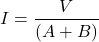This is the current passing through loop-1. And the same current will flow through resistors A and B.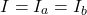The second loop is an open circuit. So, the current passing through resistor C is zero. And voltage drop of resistor C is zero. Therefore, we can neglect resistor C.

The voltage drop across the resistor B is the same as the voltage available between open circuit terminal P and Q. And the voltage drop across resistor B is,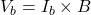This voltage is an open circuit voltage or Thevenin voltage.

## Open Circuit Voltage Test

Open circuit voltage is a potential difference between positive and negative terminals. The open-circuit voltage test is performed on battery and solar cells to identify the electrical potential capability.

The battery is used to convert chemical energy into electrical energy. And there are two types of batteries; rechargeable battery and primary battery.

Open circuit voltage test is applied to both types of batteries. And the data of this test used to calculate the state of charge (SOC) for rechargeable batteries.

Standard open circuit voltage is derived from the datasheet of the battery manufacturer. The voltage mentioned on the battery is an open-circuit voltage.

Open circuit voltage test measures the voltage of the battery when a load is not connected. So, to perform an open circuit voltage test, remove the battery if possible or take terminals for testing.

Now, set a digital multimeter on DC voltage. And measure the reading across battery terminals. This voltage is near the standard voltage. If the measured voltage is low, the battery is damaged.

For rechargeable batteries, this test is performed to check the battery is charged or discharged. In this case, a capacity test is performed to check the condition.

## Why is Voltage Not Zero on an Open Circuit?

The voltage is defined as a potential difference between two terminals. So, two points are not connected to each other and both points are connected with different voltage levels. In this condition, due to potential difference, the voltage present between two points.

Similarly, in open circuit condition, both terminals are open but it is connected with battery or other voltage sources. And both terminals of a battery are at different voltage levels.

Therefore, a potential difference is created and a voltage is present between two terminals in open circuit conditions.

## Open Circuit Voltage of a Solar Cell

In a solar cell, the maximum voltage is available at zero current condition. And this voltage is known as open-circuit voltage.

When photons hit the solar cells, the current is generated due to the bias of solar cell junctions. The open-circuit voltage is a forward bias voltage on a solar cell.

In the I-V characteristics of a solar cell, the open-circuit voltage is as shown below figure.

The equation of open circuit voltage is;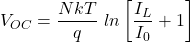Where,

I0 = Dark saturation current
IL = Light generated current
N = Ideality factor
T = Temperature
k = Boltzmann constant
q = Electronic charge

From the above equation, Voc and temperature are directly proportional. Hence, Voc increase linearly with respect to temperature. But actually, this is not happing. Because the saturation current also increases rapidly with an increase in temperature. Therefore, the effect of temperature on open circuit voltage is a complicated task. If saturation current changes with temperature changes, the open-circuit voltage decreases with temperature.

## Open Circuit Voltage Example Questions

The method to find open circuit voltage is the same as to find the Thevenin voltage. Let’s understand how to find the open-circuit voltage with an example.

### Example-1

In the above figure, load RL is connected with the DC source. Now, we disconnect the load from the source and the remaining circuit is as shown below figure.

Due to the open circuit, the current passing through the load and 10-ohm resistor is zero. And the open-circuit voltage is the same as the voltage across a 3-ohm resistor.

Apply KVL in loop-1;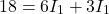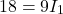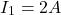Voltage across 3-ohm resistor is;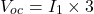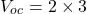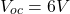### Example-2

In open-circuit condition, the load RL is disconnected from the circuit and we will find the voltage across two terminals of load. Hence, the remaining circuit after the open circuit is as below.

The open-circuit voltage is equal to the voltage across a 6-ohm resistor. So, we need to find the current passing through the 6-ohm resistor.

Now, apply KVL in outer loop;

(1)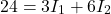We can express 3A current source in terms of loop currents.

(2)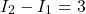Now, by solving eq-1 and eq-2, we can find the current I1 and I2. But we only need current passing through the 6-ohm resistor. And that current is I2.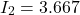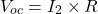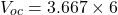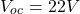Want To Learn Faster? 🎓
Get electrical articles delivered to your inbox every week.
No credit card required—it’s 100% free.